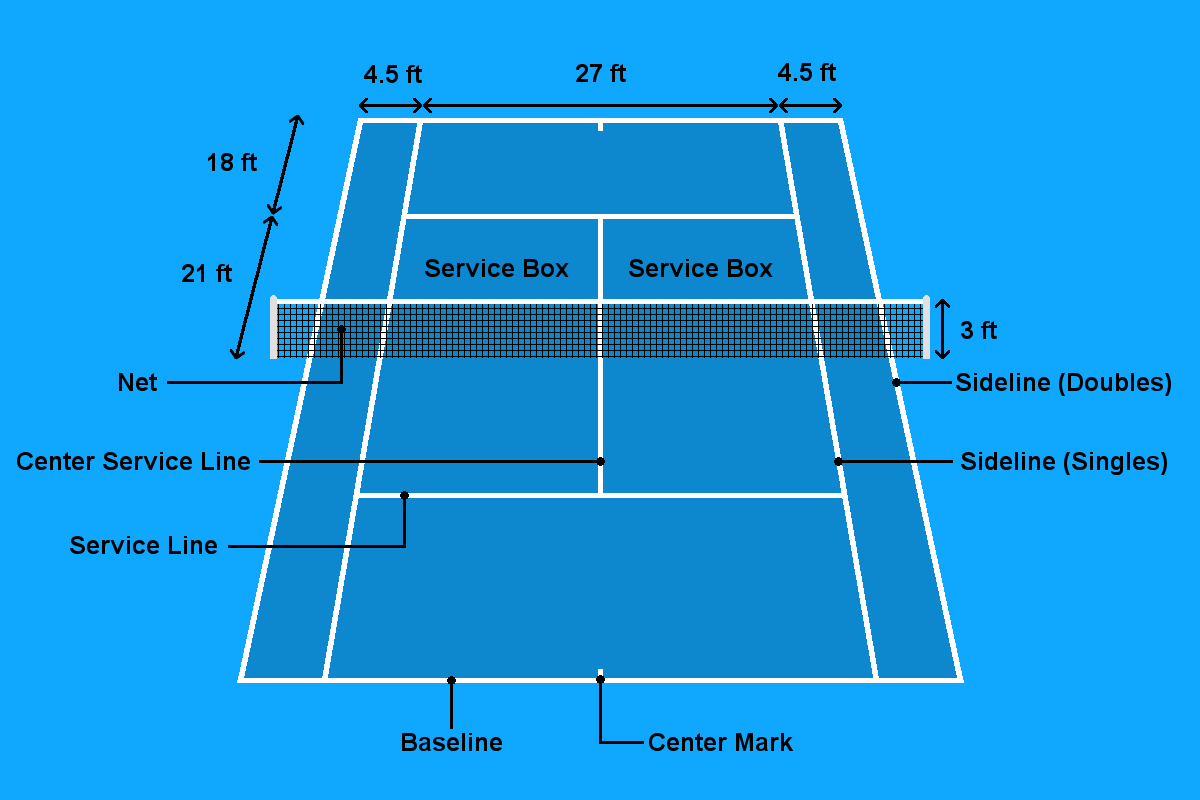# Percent Error Study Guide

INTRODUCTION

When you need to measure any item, you may not always get the measurement right the first time you measure it. Take a tennis court 🎾, for instance, where dimensions have to be accurate. The markings or lines drawn in white need to be painted with total accuracy as the slightest difference can lead to major tournament upsets! 😱

Say you and your pals were asked to measure the court, and each of you found a different measurement 🤐 That’s an error in the measurements. Subtracting the found measurement from the actual size, dividing it by the actual size, and then multiplying by 100 would net you the percent error 🏅Source

### CAN A PERCENT ERROR BE NEGATIVE?

Percent error compares the experimental figure obtained to the known or actual value. It is also known as absolute error, and the lesser it is, the closer you are to the known value.

Therefore, the difference between the experimental and the actual value is the error.

Error = Experimental figure – Known value.

When the experimental figure obtained is lower than the known value, the answer will be negative. If the experimental figure is more than the actual figure, the answer will be positive.

Therefore, Percent error may be calculated as,% Error = (Experimental figure – Known value)/Known Value X 100
For example, you are performing an experiment to calculate the density of a piece of aluminum. The known density for this metal is 2.70g/cm3. But after experimenting, you find the density to be 2. 32g/cm3, which is lower than the known figure. The percent error can be calculated as follows.

``% Error = (2.32 g/cm3 – 2.70 g/cm3) / 2.70 g/cm3 X 100 = 14.07%``

Therefore the percent error is calculated as 14.07%.

## CONCLUSION:

• If the calculated value after performing the experiment is almost close to the known value, the percent error can be zero.
• Error is usually calculated as the absolute value so that there is no room for confusion.

### FAQs:

1. Is negative percent error possible?

If the experimental figure obtained is lower than the accepted known figure, the percent error is negative. But since the final version has to be reported, the positive value is considered, so the final value cannot be negative.

2. What happens if you have a negative percent error?

Even if the experimental figure is obtained as a negative value, it is reported as the absolute value, so the final answer will not be negative.

3. Are percent errors always positive?

Percent errors are always reported as absolute values even if the experimental values are negative.

We hope you enjoyed studying this lesson and learned something cool about the Percent error! Join our Discord community to get any questions you may have answered and to engage with other students just like you! Don’t forget to download our App to experience our fun VR classrooms – we promise it makes studying much more fun! 😎

## SOURCES:

1. Percent Error. https://www.ck12.org/c/chemistry/percent-error/lesson/Percent-Error-CHEM/. Accessed 17 January 2022.
2. Percent Error. https://chem.libretexts.org/Bookshelves/Introductory_Chemistry/Introductory_Chemistry_(CK-12)/03%3A_Measurements/3.13%3A_Percent_Error. Accessed 17 January 2022.
3. Percent Error. https://www.expii.com/t/percent-error-definition-examples-4253. Accessed 17 January 2022.
4. Can Percent Error Be a Negative Number? https://www.reference.com/world-view/can-percent-error-negative-number-367cee25ac338cc4. Accessed 17 January 2022.
]]>